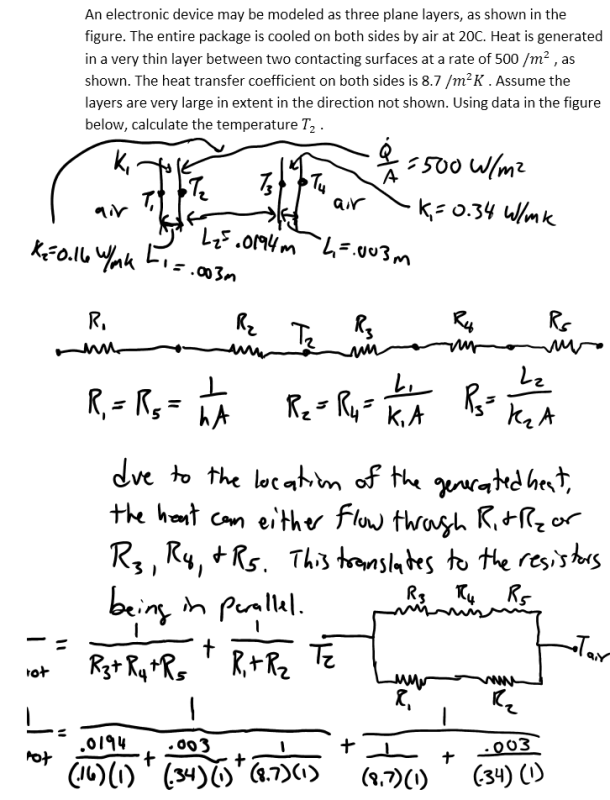heat and mass transfer problems engineering equations heat and mass transfer school homework engineering heat and mass transfer formulas heat and mass transfer solutions to heat and mass transfer problems full solution engineering problem solution heat and mass transfer math problems engineering equations heat and mass transfer school homework engineering solutions to heat and mass transfer formulas heat problems mass problem solutions to transfer problems full solution heat and mass transfer
heat and mass transfer problems engineering equations heat and mass transfer school homework engineering heat and mass transfer formulas heat and mass transfer solutions to heat and mass transfer problems full solution engineering problem solution heat and mass transfer math problems engineering equations heat and mass transfer school homework engineering solutions to heat and mass transfer formulas heat problems mass problem solutions to transfer problems full solution heat and mass transfer
Highalphabet Home Page heat and mass transfer problem solutions Heat and Mass Transfer Page
An electronic device may be modeled as three plane layers, as shown in the figure. The entire package is cooled on both sides by air at 20C. Heat is generated in a very thin layer between two contacting surfaces at a rate of 500 /m^2 , as shown. The heat transfer coefficient on both sides is 8.7 /m^2 K . Assume the layers are very large in extent in the direction not shown. Using data in the figure below, calculate the temperature T_2 .An electronic device may be modeled as three plane layers, as shown in the figure. The entire package is cooled on both sides by air at 20C. Heat is generated in a very thin layer between two contacting surfaces at a rate of 500 /m^2 , as shown. The heat transfer coefficient on both sides is 8.7 /m^2 K . Assume the layers are very large in extent in the direction not shown. Using data in the figure below, calculate the temperature T_2 .